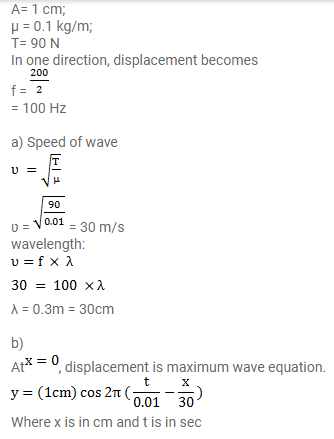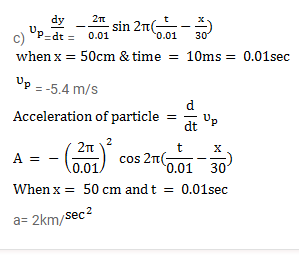# A travelling wave is produced on a long horizontal string

Question:

A travelling wave is produced on a long horizontal string by vibrating an end up and down sinusoidally. The amplitude of vibration is $1.0 \mathrm{~cm}$ and the displacement becomes zero 200 times per second. The linear mass density of the string is $0.10$ $\mathrm{kg} / \mathrm{m}$ and it is kept under a tension of $90 \mathrm{~N}$.

a. Find the speed and the wavelength of the wave

b. Assume that the wave moves in the positive $x$ direction and at $t=0$ the end $x=0$ is at its positive extreme position. Write the wave equation.

c. Find the velocity and acceleration of the particle at $x=50 \mathrm{~cm}$ at time $t=10 \mathrm{~ms}$.

Solution: